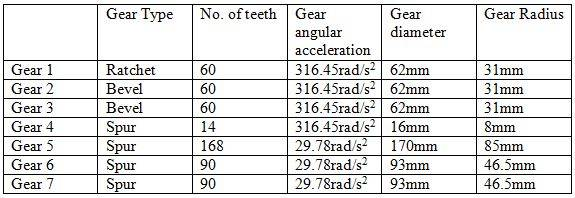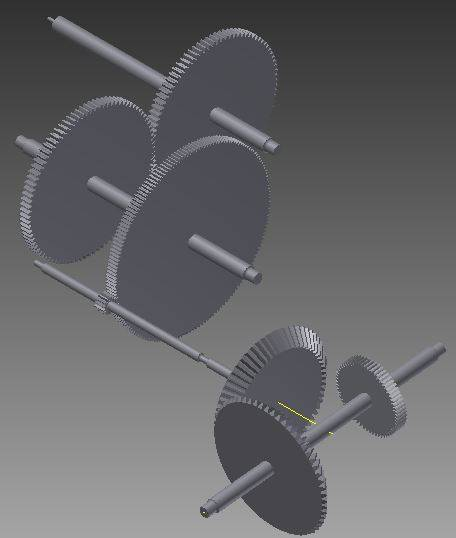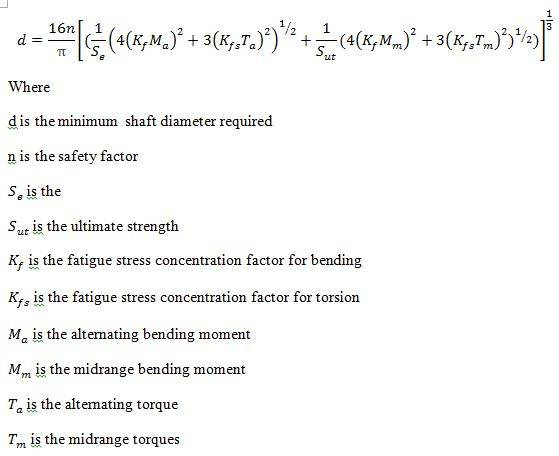# How to calculate the torque required to move the gear?

## Homework Statement

GivenWhere gear 1 is start from the bottom right gear and ends with gear 7 which is located at the top middle.The shaft length are 20cm between gear 1 and gear 2,15cm between gear 2 and gear 3 and 15cm between gear 5 and gear 6. The material of the shaft and gear are made up of acetal which density is 1410kg/m3.

May i know how to calculate the input torque required in order turn all the gear?
no load are at the end of the gear, only mass moment of inertia of the gear and the shaft need to be consider. TQ for helping.

## Homework Equations

Torque= mass moment of inertia*angular acceleration
...

## Answers and Replies

haruspex
Science Advisor
Homework Helper
Gold Member
2020 Award
Torque= mass moment of inertia*angular acceleration
...
Merely moving the gears requires no particular acceleration; it can be extremely small. As a result, there is no minimum torque required by that equation. In practice, there is a minimum torque, but that comes from static friction at the axles.

Merely moving the gears requires no particular acceleration; it can be extremely small. As a result, there is no minimum torque required by that equation. In practice, there is a minimum torque, but that comes from static friction at the axles.

if mass moment of inertia of the gear and the shaft are needed to be consider? i am required by the lecturer to do that calculationharuspex
Science Advisor
Homework Helper
Gold Member
2020 Award
I just realised you left something out. You wrote:
May i know how to calculate the input torque required in order turn all the gear?
But what you meant was
May i know how to calculate the input torque required in order turn all the gear at the specified accelerations?​
Now it makes sense. All you need to do is calculate the MI of each gear and shaft, multiply each of those by its required acceleration, and add them all up. But you cannot do that for the shafts without knowing their radii. Do they matter? I would not have thought so. The shaft lengths are irrelevant.

I just realised you left something out. You wrote:

But what you meant was
May i know how to calculate the input torque required in order turn all the gear at the specified accelerations?​
Now it makes sense. All you need to do is calculate the MI of each gear and shaft, multiply each of those by its required acceleration, and add them all up. But you cannot do that for the shafts without knowing their radii. Do they matter? I would not have thought so. The shaft lengths are irrelevant.

TQ so much.

Sorry that i miss out the acceleration and the radii of the shaft.
The acceleration is 9.81m/s2 and as the shaft is to be designed by me and no specific radii is given to it, thus the radii of the shaft i would like to use the following formula to find:As the bending moment of the shaft are negligible small, the midrange and alternating bending moment is ignored, only torsion need to be considered.
May i know how to calculate for the alternating torque, i was told by the lecturer the alternating torque is superposed but may i know why is it superposed?

I just realised the shaft length are irrelevant in the formula. Just ignore it.

Do i need to use this formula to calculate the gear torque
total torque on A to accelerate A and B :

= Ia alphaa + n2 Ib alphaa
= ( Ia + n2 Ib ) alphaa

that are found in this website:
http://www.tech.plym.ac.uk/sme/mech226/gearsys/gearaccel.htm

May i know do i implement the shaft mass moment of inertia into it?
Tq for your help!

haruspex
Science Advisor
Homework Helper
Gold Member
2020 Award
I don't know what it means for a torque to be superposed. I'm not an engineer. (I think there's another forum that would be better for that.)
I would have thought the MIs of shafts were negligible, but if you want to take them into account then you will need the lengths (to get the masses).
If you're concerned about the torque on each shaft then you will need to work backwards from the final gear calculating the torque at each step (by adding the MI*accn terms successively). The greatest torque will be nearest the power source, so in principle you could use thicker shafts there.